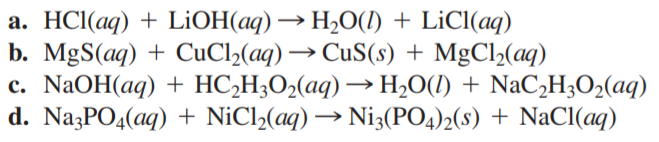×
Get Full Access to Chemistry: A Molecular Approach - 3 Edition - Chapter 4 - Problem 79e
Get Full Access to Chemistry: A Molecular Approach - 3 Edition - Chapter 4 - Problem 79e

×

# Write balanced complete ionic and net ionic equations forISBN: 9780321809247 1

## Solution for problem 79E Chapter 4

Chemistry: A Molecular Approach | 3rd Edition

• Textbook Solutions
• 2901 Step-by-step solutions solved by professors and subject experts
• Get 24/7 help from StudySoup virtual teaching assistantsChemistry: A Molecular Approach | 3rd Edition

4 5 1 353 Reviews
29
1
Problem 79E

Write balanced complete ionic and net ionic equations for each reaction.Step-by-Step Solution:
Step 1 of 3

Solution: Step1: a) The reaction between Silver nitrate(AgNO ) and Hydroc3oric acid(HCl) takes place in the following manner: AgNO (a3 + HCl(aq) -----> AgCl(aq) + HNO (aq) 3 In the above reaction, we have seen that 1 mole of AgNO requires 1 mole of HCl to 3 precipitate out all the silver ions. Now, let us calculate the number of moles of 15.0 mL of 0.200 M AgNO solution. 3 Number of moles of AgNO solution 3Volume of solution in litres X Molarity = (15/1000 L) X 0.200 M = 0.015 L X 0.200 M = 0.003 mol Thus, 0.003 mol of AgNO solution3ill require 0.003 mol of HCl to precipitate out all the silver ions. Let us calculate the volume of HCl corresponding to 0.003 mol of 0.150 M HCl solution. Volume of HCl solution in litres = Number of moles of HCl / Molarity = 0.003 mol / 0.150 M = 0.02 L Thus, 0.02 L of a 0.150 M HCl(aq) solution is needed to precipitate the silver ions from 15.0 ml of a 0.200 M AgN0 solutio3 Step2: b) The reaction between Silver nitrate(AgNO ) and potassium chloride(KCl) takes place in 3 the following manner: AgNO (a3 + KCl(aq) -----> AgCl(aq) + KNO (aq) 3 In the above reaction, we have seen that 1 mole of AgNO requires 1 mol3of KCl to precipitate out all the silver ions. Now, let us calculate the number of moles of 15.0 mL of 0.200 M AgNO solution. 3 Number of moles of AgNO solution = Volume of solution in litres X Molarity 3 = (15/1000 L) X 0.200 M = 0.015 L X 0.200 M = 0.003 mol Thus, 0.003 mol of AgNO solution3ill require 0.003 mol of KCl to precipitate out all the silver ions. Now, 1 mole of KCl = molar mass of KCl Therefore, 0.003 mol of KCl = 0.003 X molar mass of KCl = 0.003 x 74.5 g [molar mass of KCl = 74.5 g/mol] = 0.2235 g Thus, 0.2235 g of KCl is needed to precipitate the silver ions from 15.0 ml of a 0.200 M AgN0 solution. 3 Step3: c) Cost of 500 mL of 0.150 M HCl solution = $39.95 Volume of HCl used in the reaction = 0.02 L = 0.02 X 1000 mL = 20 mL Therefore, total cost of HCl used = ($39.95 / 500 mL) X 20 mL = 0.0799 $/mL X 20 mL =$1.598 Again, cost of 1 ton(907.2 Kg) of KCl = $10 Mass of KCl used in the reaction = 0.2235 g = 0.2235 / 1000 Kg = 0.0002235 Kg Therefore, total cost of KCl used = ($10 / 907.2 Kg) X 0.0002235 Kg = 0.011 $/Kg X 0.0002235 Kg =$0.0000024585 = \$2.46 x 10-6 Since, the cost of KCl is very less compared to the cost of HCl, therefore, KCl is more cost-effective.

Step 2 of 3

Step 3 of 3

##### ISBN: 9780321809247

The full step-by-step solution to problem: 79E from chapter: 4 was answered by , our top Chemistry solution expert on 02/22/17, 04:35PM. This full solution covers the following key subjects: solution, precipitate, silver, kci, add. This expansive textbook survival guide covers 82 chapters, and 9454 solutions. This textbook survival guide was created for the textbook: Chemistry: A Molecular Approach, edition: 3. Chemistry: A Molecular Approach was written by and is associated to the ISBN: 9780321809247. Since the solution to 79E from 4 chapter was answered, more than 480 students have viewed the full step-by-step answer. The answer to “?Write balanced complete ionic and net ionic equations for each reaction.” is broken down into a number of easy to follow steps, and 11 words.

Unlock Textbook Solution